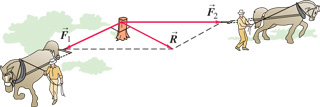# Problem: Two horses pull horizontally on ropes attached to a stump. The two forces exttip{vec {F_1}}{F_1_vec} = 1310 N and 2 that they apply to the stump are such that the net (resultant) force exttip{vec{R}}{R_vec} has a magnitude equal to that of 1 and makes an angle of 901 with 1. Find the magnitude of exttip{vec{F}_{ m 2}}{F_2_vec}.Find the direction of exttip{vec{F}_{ m 2}}{F_2_vec} (relative to exttip{vec {F_1}}{F_1_vec}).

⚠️Our tutors found the solution shown to be helpful for the problem you're searching for. We don't have the exact solution yet.

###### Problem Details

Two horses pull horizontally on ropes attached to a stump. The two forces = 1310 N and 2 that they apply to the stump are such that the net (resultant) force has a magnitude equal to that of 1 and makes an angle of 901 with 1.Find the magnitude of .

Find the direction of (relative to ).